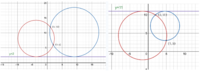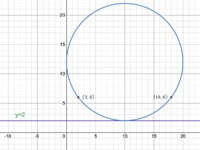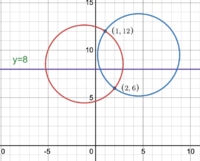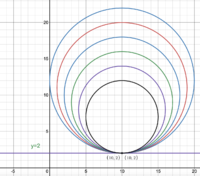# Finding the center of a cricle with two given points and a horizontal tangent.

#### toto

##### New member
I have posted this on stackoverflow and the answer i got is correct but i am unable to understand some part of it, here is the answer:
INPUT:
two given points:
[x1, y1]
[x2, y2]
and a real number:
c
where y = c is a horizontal line

CALCULATION of the coordinates of the centre of the circle
that passes through the two given points [x1, y1] and [x2, y2]
and is tangent to the horizontal line y = c:

k = (x2 - x1) / (y2 - y1)
x_P = x1 + k * (c - y1)
t = sqrt((x_P - x1)^2 + (c - y1)^2) * math.sqrt((x_P - x2)^2 + (c - y2)^2)
t = sqrt(t)
x_center = x_P - t * k / abs(k)
y_center = (y1 + y2) / 2 - k * (x_center - (x1 + x2)/2)
what I am unable to understand is the variable t, what is t? and how is he finding the x_center and y_center?

#### lex

##### Full Member
•toto

#### Dr.Peterson

##### Elite Member
•toto and pka

#### toto

##### New member
Here is the result of that construction:

View attachment 26868

There's one sign error (in the definition of x_center).

Are you familiar with the fact that AB*BP = TP^2? That's what t is.
x_center has two solutions actually, as I am implementing fortunes algorithm the solution which is closer to AB line is the ideal solution.
thank you for the answer

#### toto

##### New member
They're using the 'power of a point theorem':
View attachment 26865

View attachment 26866

Their 't' is PT in this picture.
So, it's obvious how they get x_center.
To get y_centre, they use the fact that the perpendicular line through the midpoint of the chord MN, goes through the centre of the circle.
thank you I got it!

#### Dr.Peterson

##### Elite Member
Are you familiar with the fact that AB*BP = TP^2? That's what t is.
I didn't notice my typo until now. That should be AP*BP = TP^2 .

#### lex

##### Full Member
Of course this method doesn't work when $$\displaystyle y_1=y_2$$.
You'll need another method for that.

#### lex

##### Full Member
Here is the result of that construction:
There's one sign error (in the definition of x_center).
Are you familiar with the fact that AB*BP = TP^2? That's what t is.
x_center has two solutions actually, as I am implementing fortunes algorithm the solution which is closer to AB line is the ideal solution.
thank you for the answer
Yes, when this method is applicable, there are two solutions. The ABS(k) bit isn't necessary - when there are two solutions, just add and subtract t, to get the two x_center values. (That is, if you want the 2 solutions, of course).

Two examples with 2 solutions each:An example with 1 solution (when the two points are on the same horizontal level).
(When there is only one solution, the method used in this post doesn't work - an alternative is needed).An example with 0 solutions:An example with an infinite number of solutions!Last edited: